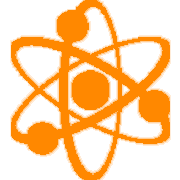#### SCI-HS.PS2.01High School (SCI) Physical Science Standards
[PS2] Motion and Stability: Forces and Interactions

##### SCI-HS.PS2.01 Analyze data to support the claim that Newton’s second law of motion describes the mathematical relationship among the net force on a macroscopic object, its mass, and its acceleration.

Clarification Statement: Physical Science and Physics: Examples of data could include tables or graphs of position or velocity as a function of time for objects subject to a net unbalanced force, such as a falling object, an object rolling down a ramp, or a moving object being pulled by a constant force in one dimension.
Disciplinary Core Ideas
PS2.A: Forces and Motion Newton’s second law accurately predicts changes in the motion of macroscopic objects.

## Student Learning Targets:

• I can

• I can

• I can

### Product Targets

• I can

Student "I can" statements are embedded within the proficiency scale.

## Proficiency Scale (Physical Science)

 Score Description Sample Activity 4.0 In addition to Score 3.0, the student demonstrates in-depth inferences and applications regarding more complex material that go beyond end of instruction expectations. Themselves as an individual (myself, my family, my friends) Our society (environment, economy, infrastructure) Our culture (beliefs, norms, people) Our species (mankind, global, environment) - 3.5 In addition to Score 3.0 performance, the student demonstrates in-depth inferences and applications regarding the more complex content with partial success. 3.0 “The Standard.” The student demonstrates no major errors or omissions regarding any of the information and processes that were end of instruction expectations. The student will be able to (Motion): Solve problems related to time, distance, displacement, speed and velocity.         Explain the relationship between motion and a frame of reference. Calculate problems related to time, distance, displacement, speed, and velocity Graph velocity on a distance-time graph. Calculate acceleration as the rate at which velocity changes. Graph acceleration on a velocity-time graph. Explain the effects of unbalanced forces on the motion of objects· Compare and contrast static and kinetic friction Identify ways friction may be either helpful or harmful.   The student will be able to (Work and Energy): Calculate the work done on an object and the rate at which work is done       Calculate the MA of various machines      Calculate KE and GPE Distinguish between mechanical and non-mechanical energy Explain the Law of conservation of energy      Analyze the efficiency of machines Define work and power Name and describe the six types of simple machines       Define potential energy and kinetic energy Identify examples of energy transformations - 2.5 The student demonstrates no major errors or omissions regarding the simpler details and processes (Score 2.0 content) and partial knowledge of the more complex ideas and processes (Score 3.0 content). 2.0 The student demonstrates no major errors or omissions regarding the simpler details and processes but exhibits major errors or omissions regarding the more complex ideas and processes (Score 3.0 content). The student will be able to define (Motion): Acceleration, Average speed, Constant velocity, Force, Frame of reference, Motion, Net force, Newton, Speed, Unbalanced force, Velocity The student will be able to define (Work and Energy): Compound machine, Energy, Fulcrum, Mechanical advantage, Power, Watt However, the student exhibits major errors or omissions regarding the more complex ideas and processes. - 1.5 The student demonstrates partial knowledge of the simpler details and processes (Score 2.0 content) but exhibits major errors or omissions regarding the more complex ideas and procedures (Score 3.0 content). 1.0 With help, the student demonstrates a partial understanding of some of the simpler details and processes (Score 2.0 content) and some of the more complex ideas and processes (Score 3.0 content). - 0.5 With help, the student demonstrates a partial understanding of some of the simpler details and processes (Score 2.0 content) but not the more complex ideas and processes (Score 3.0 content). 0.0 Even with help, the student demonstrates no understanding or skill. -

Websites

Vocabulary